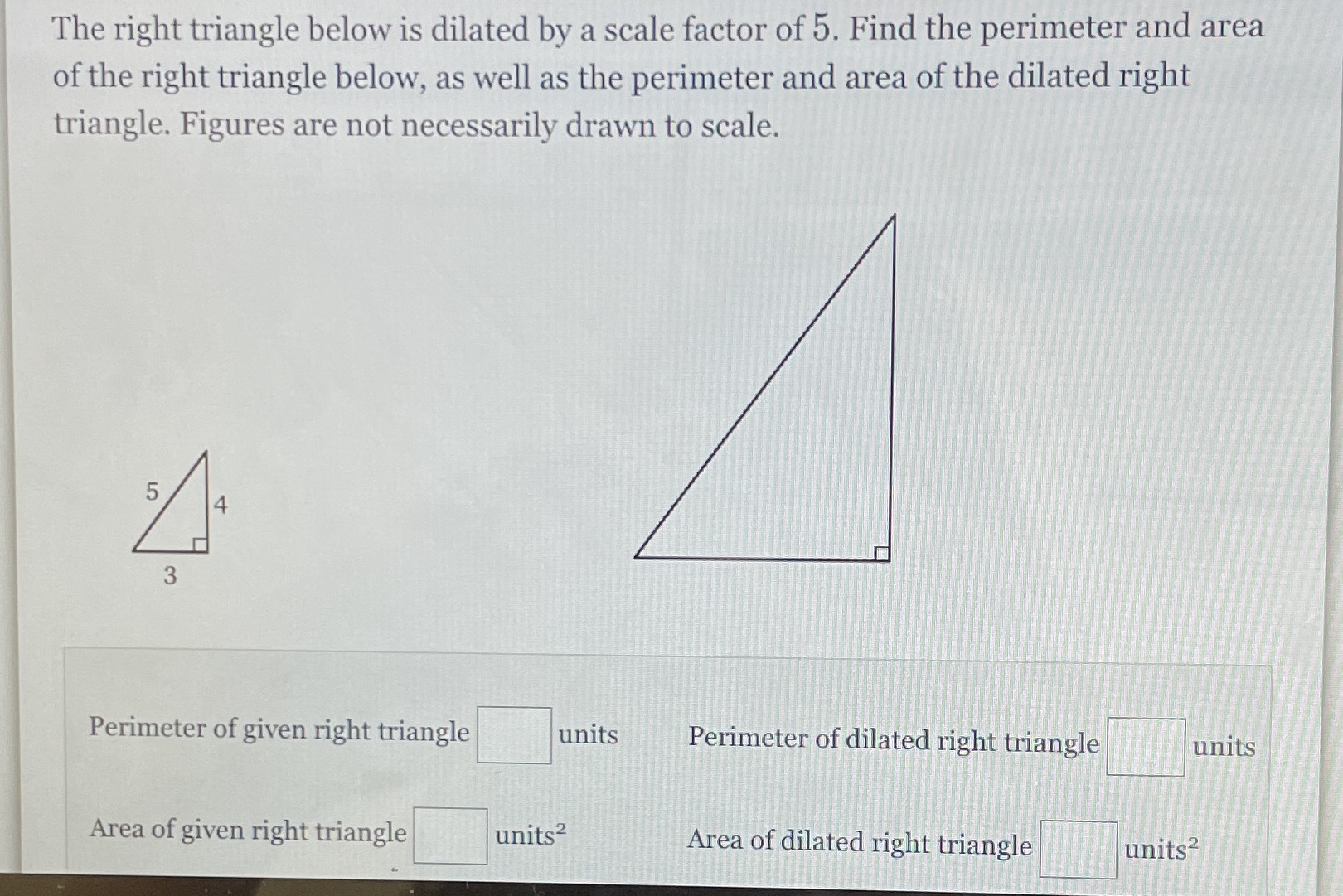### Still have math questions?

Trigonometry
QuestionThe right triangle below is dilated by a scale factor of $$5 .$$ Find the perimeter and area of the right triangle below, as well as the perimeter and area of the dilated right triangle. Figures are not necessarily drawn to scale.

a. 12

b. 10

c. perimeter Dilated triangle = 60

d. Area = 150cm

Solution
View full explanation on CameraMath App.